# Oberseminar Analysis Winter 2012/13

## Organizers: B. Zwicknagl, S. Conti, H. Koch, S. Müller, B. Niethammer, M. Rumpf, B. Schlein, C. Thiele, J. López-Velázquez

• Thursday, October 18, 2012, 2:15 p.m., seminar room 2.040
André Schlichting
The Eyring-Kramers formula for Poincaré and logarithmic Sobolev inequalities

We consider a class of Fokker-Planck equations in the regime of low
temperature. The presented technique provides a new proof for the
sharp estimate of the spectral gap of the associated generator,
which has the variational formulation as the optimal constant
in the Poincaré inequality. Moreover, this approach generalises to
the optimal constant in the logarithmic Sobolev inequality, which has
no characterisation by the spectrum of the generator. This sharp
identification is called Eyring-Kramers formula and allows to obtain
fine properties of the relaxation behaviour of the associated diffusion
process. The result follow from two main ingredients: The first one uses
an explicit construction of a Lyapunov function for the dynamics restricted
to a basin of attraction resulting in "good" Poincaré and log-Sobolev constants.
This mimics the fast convergence of the diffusion to metastable states.
The second ingredient is a mean-difference estimate, where we propose a
dynamic interpretation of the negative Sobolev norm as weighted transport
distance. Therewith, we obtain the main contribution to the Poincaré and
logarithmic Sobolev constant, resulting from exponential long waiting times
of jumps between metastable states of the diffusion. (joint work with Georg Menz)

• Thursday, November 15, 2012, 2:15 p.m., seminar room 2.040
Martijn Zaal (Vrije Universiteit Amsterdam)
Gradient flows in metric and non-metric spaces and applications to parabolic free
boundary problems

In this talk, a very simple model for cell swelling by osmosis will be studied.
The problem consists of diffusion in a free domain. The boundary moves by
surface tension and osmotic force. A variational formulation for this problem
will be introduced, and it will be shown that the osmotic force can be thought
of as a Lagrange multiplier. Finally, it appears that the concept of gradient
flow can be stretched a bit further than metric spaces, and that this
generalization is useful to study the variational structure of the mean
curvature flow.

• Thursday, November 22, 2012, 2:15 p.m., Lipschitz-Saal (room 1.016)
Marcello Lucia (City University of New York)
Best constant in some Onofri type inequalities
In 1982 Onofri derived on the two-dimensional sphere an optimal inequality
for a Moser-Trudinger type functional. The aim of this talk is to present
similar results either on closed surfaces, or on a disk within the class of
functions that are invariant under the action of a group of rotations. Those
results can be applied to classify the steady states of a chemotaxis system.

• Thursday, November 29, 2012, 2:15 p.m., seminar room 2.040
Allan Chan
Energyscaling, domain-branching and SO(2)-invariance in solid-solid phase transitions
In the early 90s Kohn and Müller established a model for the formation
of domain pattern in martensitic shape-memory alloys. For a vectorial
generalization of their model for solid-solid phase transitions with SO(2)
invariance, we discuss the cases with one and two rank-1 connections and
derive upper and lower bounds to the infimum energy with domain branching.

• Thursday, December 6, 2012, 2:15 p.m., Lipschitz-Saal (room 1.016)
Yukihiro Seki
Multiple peak aggregations for the Keller-Segel system
We discuss two-dimensinal Keller-Segel system, a classical model of chemotactic
aggregation.
We derive matched asymptotic expansions for a solution whose amount of
mass aggregation is 8N\pi, where N = 1, 2, 3, ...
Previously available asymptotics had been computed only for the case of N = 1.
This talk is based on a joint work with J.J.L. Vela\'{a}zquez and Y. Sugiyama.

• Thursday, December 6, 2012, 3:30 p.m., Lipschitz-Saal (room 1.016)
Manuel Gnann (MPI MIS, Leipzig)
Viscous thin fi lms: towards a regularity theory for the moving contact line
Abstract

• Thursday, January 10, 2013, 2:15 p.m., seminar room 2.040
Cyrill Muratov (New Jersey Institute of Technology)
On shape of charged drops: an isoperimetric problem with a competing non-local term.
In this talk I will give an overview of my recent work with H. Knuepfer
on the analysis of a class of geometric problems in the calculus of variations.
I will discuss the basic questions of existence and non-existence of energy
minimizers for the isoperimetric problem with a competing non-local term.
A complete answer will be given for the case of slowly decaying kernels in
two space dimensions, and qualitative properties of the minimizers will be
established for general Riesz kernels.

• Thursday, January 10, 2013, 3:30 p.m., seminar room 2.040
Dhia Mansour (Tuebingen University)
Variational discretization of linear wave equations on evolving surfaces
We introduce a linear wave equation on a moving surface derived from the
Hamilton's  principle of stationary action. The variational principle is discretized
with functions that are piecewise linear in space and time. This yields a
discretization of the wave equation in space by evolving surface finite elements
and in time by a variational integrator, a version of the leapfrog or
Stoermer--Verlet method. Stability and convergence of the full discretization
in the natural time-dependent norms  are analyzed. Under suitable assumptions,
we obtain optimal-order error estimates. Numerical experiments illustrate the
behavior of the fully discrete method.
The talk is based on joint work with Ch. Lubich (Tuebingen).

• Thursday, January 17, 2013, 2:15 p.m., Lipschitz-Saal (room 1.016)
Michal Wrochna (Georg-August-Universität Göttingen)
Positive-frequency solutions of the Klein-Gordon equation: the case of
overcritical potentials

In quantum field theory on curved space-time or in external potentials, a
central problem is to find solutions of the underlying Klein-Gordon equation
with a prescribed singularity structure. I will explain the original motivation
and modern formulations of the problem in terms of the wave front set, and
I will present some recent constructions. This will include the analysis of the
`overcritical case', in which the quantities preserved by the evolution are
non-positive. As an outlook, I will comment on perspectives in systems with
Coulomb potential.
• Thursday, January 17, 2013, 3:30 p.m., Lipschitz-Saal (room 1.016)
Benedikt Wirth (New York University)
A convex approximation of Euler's elastica functional
Euler's elastica energy has often been proposed for curvature regularization
in image processing, however, global minimizers are difficult to obtain due to
the high nonlinearity and nonconvexity. We introduce a convex, lower
semi-continuous, coercive approximation, which is thus very well-suited as a
regularizer in image processing. The approximation is closely related to the
exact convex relaxation (which seems very difficult to understand). Interestingly,
the exact convex relaxation of the elastica energy reduces to constantly zero
if the total variation part of the elastica energy is neglected. The convex
approximation arises via functional lifting of the image gradient into a Radon
measure on the four-dimensional space $\Omega \times S^1 \times \mathbb{R}$,
$\Omega \subset \mathbb{R}^2$, of which the first two coordinates
represent the image domain and the last two the normal and curvature of the
image level lines. It can be expressed as a linear program that can robustly be
solved on the GPU after discretizing the involved Radon measures via a linear
combination of line measures concentrated on curve segments (joint work with
Kristian Bredies and Thomas Pock).
• Thursday, January 24, 2013, 2:15 p.m., seminar room 2.040
Marcello Porta
Many-body interactions in graphene
In this talk I will discuss models for interacting fermions on the honeycomb lattice.
The goal is to understand the thermodynamic properties of graphene, a newly
discovered material which can be considered as the first realization of a
(quasi-) 2d crystal. In the case of weak short range interactions I will present
a theorem proving the universality of the conductivity, in the limit of zero
temperature and zero frequency. Then, I will briefly discuss the effect of long
range electromagnetic interactions; in this case, anomalous (i.e. interaction
dependent) exponents appear in the perturbative expansion of the correlations.
All results are obtained using rigorous Renormalization Group methods.
In both cases, a crucial role is played by gauge symmetry and Ward identities.
This is joint work with A. Giuliani and V. Mastropietro.

• Wednesday, February 2, 2013, 2:00 p.m., seminar room 1.007
Tuesday, March 5, 2013, 2:15 p.m., seminar room 1.007
Wednesday, March 6, 2013, 2:15 p.m., seminar room 1.007
Alexander Volberg (Michigan State)
On a problem of David and Semmes in a co-dimension one case

• Thursday, March 7, 2013, 2:15 p.m., seminar room 1.007
Paco Villarroya, University of Lund (Sweden)
A new T(1) Theorem for multiparameter singular integrals
In this talk, we present a new T(1) Theorem
characterizing the boundedness of singular integral
operators on product spaces. Our different approach avoids
the use of vector-value Calderon-Zygmund theory and so,
it does not require the assumption of boundedness of the
operator when restricted to the lower parameter case. This
represents an improvement of a classical result of J.L. Journe,
which is still regarded as the best extension of Calderon-Zygmund theory
to the product setting. As an application, we obtain a stronger version
of a classical theorem of R. Fefferman and E. Stein about non-isotropic
convolution operators.

• Thursday, March 28, 2013, 2:15 p.m., Lipschitz-Saal (room 1.016)
Alexander Volberg (Michigan State)
Bellman functionals and weighted problems
We show how Bellman functions of infinite number of variables appear
naturally and help to solve some problems concerning the weighted estimates
of Singular Integrals.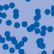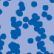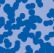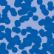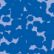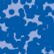## News

Der SFB 1060 Die Mathematik der emergenten Effekte hat eine dritte Förderperiode erhalten. (26.11.20)

Prof. Dr. Andreas Eberle erhält den diesjährigen Lehrpreis der Universität Bonn. (22.07.2020)

Herr Dr. Richard Höfer erhielt den Hausdorff-Gedächtnispreis 2019 der Fachgruppe Mathematik für die beste Disseration. Betreut wurde die Arbeit von Prof. J. Velázquez (29.01.2020).

Einer der Preise der Bonner Mathematischen Gesellschaft (BMG) für die besten Bachelorarbeiten 2019 ging an Herrn Peter Holderrieth unter der Betreuung von Prof. A. Eberle (29.01.2020).

Contact

Managing Director: Prof. Dr. Massimiliano Gubinelli